# Just Deep Is too Flat: Exploring New Dimensions With HyperNets

### A developer and AI expert asks whether the formalism of neural networks can be extended in some generally useful way, and explores one option.

· AI Zone · Tutorial
Save
4.34K Views

## Introduction

“In summary, a feedforward network with a single layer is sufficient to represent any function, but the layer may be infeasibly large and may fail to learn and generalize correctly." - Ian Goodfellow, DLB

A remarkable aspect of artificial neural networks, often unknown to the general public, is that they are universal approximators. They can compute any function. Since nearly every process can be considered as a form of function computation, this characteristic of artificial neural networks is the reason they are so versatile.

Artificial neural networks are essentially a way to represent functions in a certain basis. Also, they are not unique in being universal approximators. That characteristic is also present, amongst others, in polynomials and harmonic functions. What differentiates the neural networks from polynomials in machine learning is their ability to hierarchically represent and approximate basis functions on their own.

Despite their impressive characteristics, neural networks still possess some fundamental limitations. In one of our previous research articles, we concluded that although neural networks don’t just memorize patterns and do show some form of generalization, they fail to learn (generalize/extrapolate) invariants in discriminative settings or transformations in generative settings.

Therefore, it is natural to wonder whether the formalism of neural networks can be extended in some generally useful way?

## HyperNets

One option is to make neural networks not just deep yet plain, but higher-order function representations. In other words, we introduce connections on connections.

This idea is far from new: one can think about the higher-order functions in functional programming, or higher-order predicate calculus, or consider the representation of different dynamic systems as neural networks.

Hierarchical Bayesian Modeling, for instance, supposes not just a hierarchy of layers but also a hierarchy of models; each meta-model specifies a prior distribution over parameters (e.g., network weights) of a lower-level model.

In fact, this extension is also possible neurophysiologically. The idea that glial cells can modulate synaptic conductivity is rather old, and artificial neuro-glial networks have already been researched extensively.

We propose to unite all of these related concepts under the term HyperNets (this term was used in an earlier work, but in a narrower sense). We use the term HyperNets to refer to artificial neural networks that have higher-order connections, i.e., connections on connections.

So why are HyperNets not being widely used? We believe that this might be due to a lack of understanding of their benefits, and due to the absence of their general use cases. In the next section, we will try to address these issues.

## Advantages and Use Cases of HyperNets

In a previous research article, we discussed the following problem:

Let's take a function f (x, a), which has x as input and a as parameters. For example, f can return a rotated version of image x by an angle a. And we want to learn (or, at least, approximate) this function by neural networks from input-output examples.

Can neural networks achieve the stated goal? Certainly. Especially the deep and large neural networks. However, the neural networks will have some substantial limitations.

It has been observed that if the training set contains examples only for a limited range of a, then the Deep Neural Networks (DNNs) fail to accurately generalize outside of that range (with some exceptions). This limitation explains why DNNs require massive representative training sets covering the whole space of parameters of a function to be approximated.

For example, let’s say we train a DNN to rotate MNIST digits within a specific range of angles. This DNN will fail to rotate other images (especially, non-MNIST images) by angles that lie outside of its trained range.

Therefore, it is accurate to say that the DNN fails to learn, or generalize, the function of rotation itself. Rather, it approximates the function (memorizes with interpolation) within a given range.

One thing to note is that DNNs can easily learn to rotate images to any fixed degree. However, in such scenarios, they learn different weights for each rotation angle.

This scenario presents us with a general use case for HyperNets:

If we have a function f (x, a) with heterogeneous arguments (or a parameterized family of functions), then instead of approximating this function directly, one can try to approximate a higher-order function g (a) that returns a one-argument function corresponding to the projection of f onto given a, i.e. (g (a))(x)=f (x, a). This technique is known as Currying and is a reference to the logician Haskell Curry.

Thus, instead of approximating f (x, a) with some DNN(x, a | w), we can try to construct a HyperNet in the form DNN(x | w=DNN(a | w’)).

But will this method work? We studied this question in our recent paper on the example of learning image transformations.

## Learning Transformations

As a baseline model, we used an autoencoder (we considered both convolutional and fully-connected), in which the decoder also received the transformation parameters to be applied to the image (e.g., sine and cosine of the rotation angle).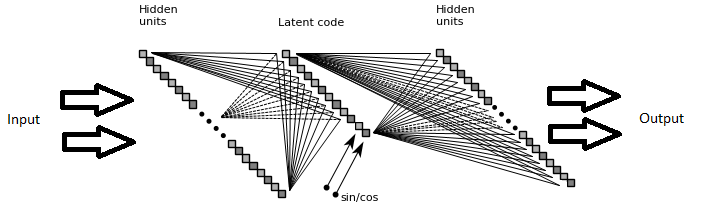The Autoencoder

The architecture of the HyperNet was similar, but the transformation parameters were fed to the higher-level control network, which influenced the connection weights of the autoencoder. In the simplest case, this “autoencoder” did not even have hidden layers: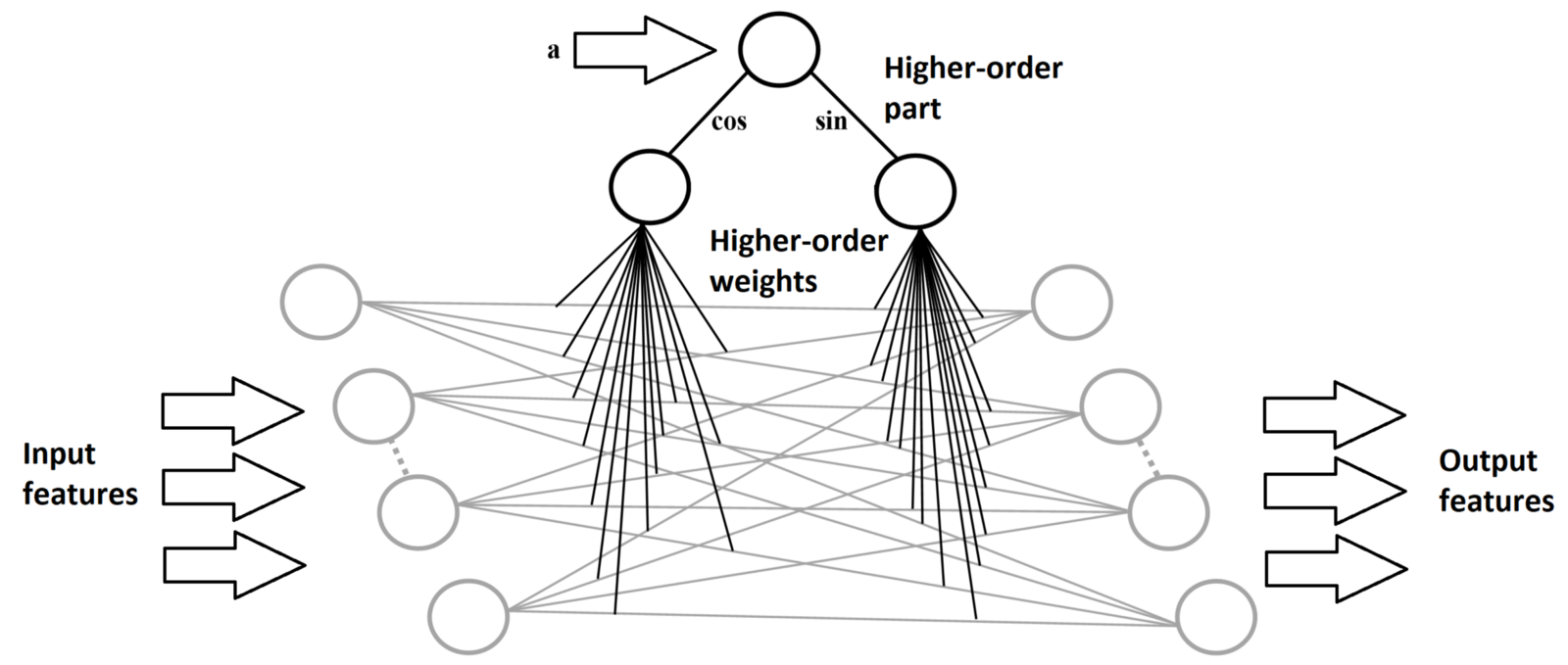Simplest HyperNet for learning image rotation

Just like the models mentioned in the previous research article, we trained our models to rotate all of the MNIST digits by all angles, but for the numbers “4” and “9” the models were trained only within the limited range of [–45ᵒ,45ᵒ]. The result of reconstruction depending on the rotation angle for ‘4’ is shown below.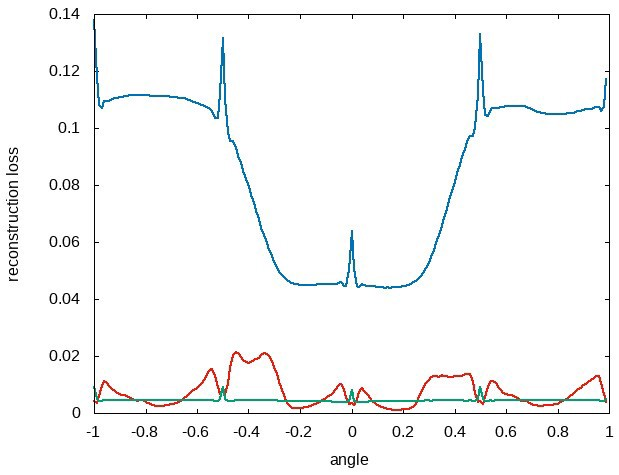Reconstruction loss for autoencoders (blue), shallow HyperNet (red) and deep HyperNet (green)

It can be seen from the results that the HyperNets learn to transfer rotation for all angles almost perfectly. In fact, the HyperNet model trained on the MNIST digits managed to transfer the learned ability to rotate images to non-MNIST symbols (pairs of columns correspond to the ideal result and what is produced by the network):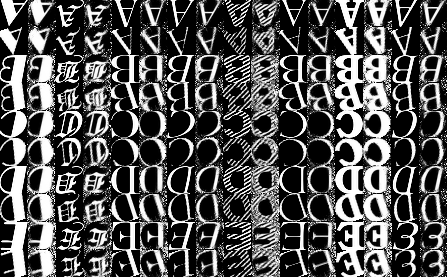The results of rotation of novel images

Therefore, it can be stated that the HyperNets actually learn the transformation procedure decoupled from the image content.

We would also like to point out that no model of image transform was built into the network. In fact, the same architecture, without any changes and additional prior information, except for accepting a larger vector of transformation parameters, can learn to apply affine transformation with excellent results: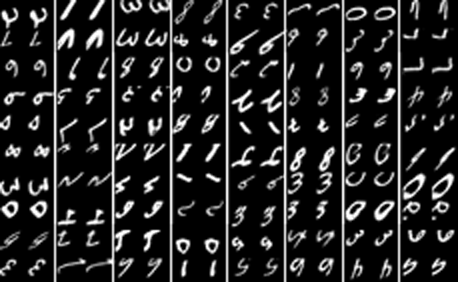Successful application of the learned affine transform

Further details of the models and experiments can be found in our research paper, and the code is available in this repository of SingularityNET’s GitHub.

## Compensation of Transformations

Based on the details that we have provided, one criticism of HyperNets might be that the task of training a model to perform an image transformation, given its parameters, is too contrived. However, nothing prevents us from feeding the HyperNet’s higher-level control network with the image itself and stating the task to normalize the image (e.g., to produce its non-rotated version without knowing the rotation angle).

The following figure shows rotated digits and the result of their normalization by the trained HyperNet model. Interestingly, the model somehow learns to distinguish rotated ‘6’ and ‘9’ (although it was not always correct).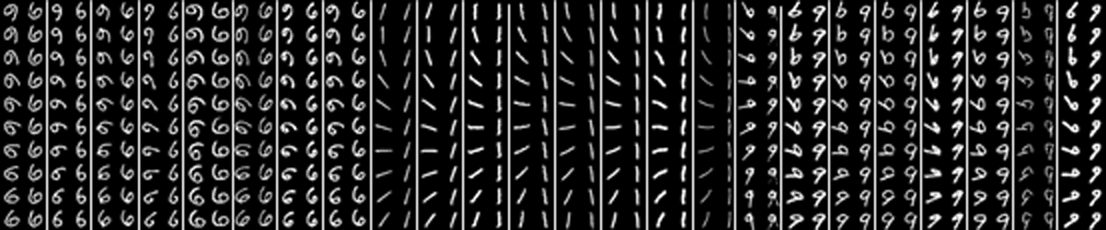Rotation compensation without a known angle

We understand that Spatial Transformers can also solve this task. However, the substantial difference is that Spatial Transformers rely on the known model of spatial transformation, this might be more practical in some instances, but it will be much less general.

We would like to point out that this HyperNet model was also able to transfer the ability to compensate rotation to novel images. For comparison purposes, if we train autoencoders and HyperNets showing the number ‘4’ in the range [–45ᵒ,45ᵒ] and then check the reconstruction loss of the normalized digit for all the angles, we will get: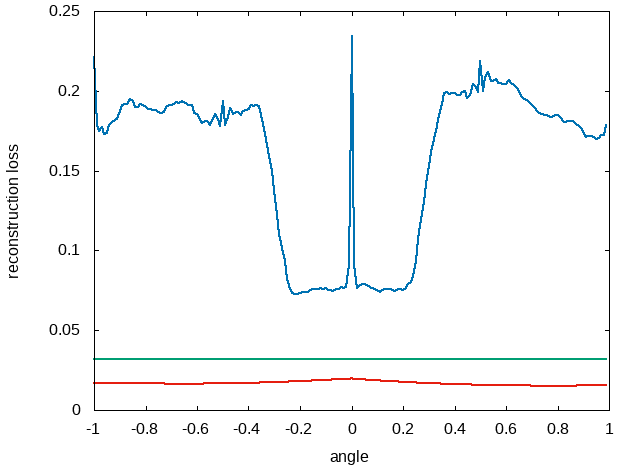Reconstruction loss for autoencoders (blue), shallow HyperNet (red), and deep HyperNet (green)

## What’s Next?

We hope it is clear that if we want to approximate a function of two heterogeneous arguments or a parameterized family of functions, HyperNets as approximations of higher-order functions (naturally appearing when we curry functions of multiple arguments) can be useful.

In this article, we looked at just one example of HyperNets application to learning to apply/compensate for spatial transformations. Moving forward, to highlight the generality of the approach, we will describe how HyperNets can be used to generalize the adaptive dropout or to implement an active vision for a Visual Question Answering task.

In the future, we plan to use HyperNets to populate the SingularityNET platform with agents possessing original and extended functionalities.

Topics:
artificial intelligence, neural nets, neural network, ai and machine learning, machine learning

Published at DZone with permission of Alexey Potapov.

Opinions expressed by DZone contributors are their own.